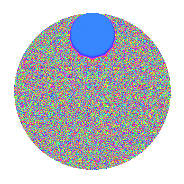# Properties

 Label 2.84.a.bLevel 2 Weight 84 Character orbit 2.a Self dual Yes Analytic conductor 87.254 Analytic rank 1 Dimension 3 CM No Inner twists 1

# Related objects

## Newspace parameters

 Level: $$N$$ = $$2$$ Weight: $$k$$ = $$84$$ Character orbit: $$[\chi]$$ = 2.a (trivial)

## Newform invariants

 Self dual: Yes Analytic conductor: $$87.2544256533$$ Analytic rank: $$1$$ Dimension: $$3$$ Coefficient field: $$\mathbb{Q}[x]/(x^{3} - \cdots)$$ Coefficient ring: $$\Z[a_1, \ldots, a_{5}]$$ Coefficient ring index: $$2^{24}\cdot 3^{11}\cdot 5^{2}\cdot 7$$ Fricke sign: $$-1$$ Sato-Tate group: $\mathrm{SU}(2)$

## $q$-expansion

Coefficients of the $$q$$-expansion are expressed in terms of a basis $$1,\beta_1,\beta_2$$ for the coefficient ring described below. We also show the integral $$q$$-expansion of the trace form.

 $$f(q)$$ $$=$$ $$q +2199023255552 q^{2} +(-5621858992801999908 - \beta_{1}) q^{3} +$$$$48\!\cdots\!04$$$$q^{4} +(-$$$$29\!\cdots\!90$$$$+ 138290647 \beta_{1} - 1657 \beta_{2}) q^{5} +(-$$$$12\!\cdots\!16$$$$- 2199023255552 \beta_{1}) q^{6} +($$$$24\!\cdots\!96$$$$- 274375425188998 \beta_{1} - 1240461740 \beta_{2}) q^{7} +$$$$10\!\cdots\!08$$$$q^{8} +($$$$96\!\cdots\!37$$$$- 14493938576442927174 \beta_{1} + 56303458286490 \beta_{2}) q^{9} +O(q^{10})$$ $$q +2199023255552 q^{2} +(-5621858992801999908 - \beta_{1}) q^{3} +$$$$48\!\cdots\!04$$$$q^{4} +(-$$$$29\!\cdots\!90$$$$+ 138290647 \beta_{1} - 1657 \beta_{2}) q^{5} +(-$$$$12\!\cdots\!16$$$$- 2199023255552 \beta_{1}) q^{6} +($$$$24\!\cdots\!96$$$$- 274375425188998 \beta_{1} - 1240461740 \beta_{2}) q^{7} +$$$$10\!\cdots\!08$$$$q^{8} +($$$$96\!\cdots\!37$$$$- 14493938576442927174 \beta_{1} + 56303458286490 \beta_{2}) q^{9} +(-$$$$65\!\cdots\!80$$$$+$$$$30\!\cdots\!44$$$$\beta_{1} - 3643781534449664 \beta_{2}) q^{10} +(-$$$$83\!\cdots\!88$$$$+$$$$13\!\cdots\!49$$$$\beta_{1} - 78240138957300760 \beta_{2}) q^{11} +(-$$$$27\!\cdots\!32$$$$-$$$$48\!\cdots\!04$$$$\beta_{1}) q^{12} +(-$$$$44\!\cdots\!58$$$$+$$$$16\!\cdots\!23$$$$\beta_{1} +$$$$36\!\cdots\!35$$$$\beta_{2}) q^{13} +($$$$53\!\cdots\!92$$$$-$$$$60\!\cdots\!96$$$$\beta_{1} -$$$$27\!\cdots\!80$$$$\beta_{2}) q^{14} +(-$$$$51\!\cdots\!80$$$$+$$$$85\!\cdots\!74$$$$\beta_{1} +$$$$44\!\cdots\!56$$$$\beta_{2}) q^{15} +$$$$23\!\cdots\!16$$$$q^{16} +($$$$49\!\cdots\!66$$$$+$$$$77\!\cdots\!78$$$$\beta_{1} +$$$$74\!\cdots\!30$$$$\beta_{2}) q^{17} +($$$$21\!\cdots\!24$$$$-$$$$31\!\cdots\!48$$$$\beta_{1} +$$$$12\!\cdots\!80$$$$\beta_{2}) q^{18} +($$$$18\!\cdots\!00$$$$-$$$$10\!\cdots\!09$$$$\beta_{1} +$$$$28\!\cdots\!40$$$$\beta_{2}) q^{19} +(-$$$$14\!\cdots\!60$$$$+$$$$66\!\cdots\!88$$$$\beta_{1} -$$$$80\!\cdots\!28$$$$\beta_{2}) q^{20} +($$$$12\!\cdots\!32$$$$+$$$$98\!\cdots\!68$$$$\beta_{1} +$$$$54\!\cdots\!40$$$$\beta_{2}) q^{21} +(-$$$$18\!\cdots\!76$$$$+$$$$29\!\cdots\!48$$$$\beta_{1} -$$$$17\!\cdots\!20$$$$\beta_{2}) q^{22} +(-$$$$26\!\cdots\!68$$$$-$$$$24\!\cdots\!86$$$$\beta_{1} -$$$$37\!\cdots\!60$$$$\beta_{2}) q^{23} +(-$$$$59\!\cdots\!64$$$$-$$$$10\!\cdots\!08$$$$\beta_{1}) q^{24} +(-$$$$16\!\cdots\!25$$$$+$$$$16\!\cdots\!60$$$$\beta_{1} -$$$$98\!\cdots\!60$$$$\beta_{2}) q^{25} +(-$$$$98\!\cdots\!16$$$$+$$$$36\!\cdots\!96$$$$\beta_{1} +$$$$79\!\cdots\!20$$$$\beta_{2}) q^{26} +($$$$88\!\cdots\!20$$$$+$$$$93\!\cdots\!22$$$$\beta_{1} -$$$$94\!\cdots\!60$$$$\beta_{2}) q^{27} +($$$$11\!\cdots\!84$$$$-$$$$13\!\cdots\!92$$$$\beta_{1} -$$$$59\!\cdots\!60$$$$\beta_{2}) q^{28} +($$$$12\!\cdots\!10$$$$+$$$$43\!\cdots\!27$$$$\beta_{1} -$$$$38\!\cdots\!85$$$$\beta_{2}) q^{29} +(-$$$$11\!\cdots\!60$$$$+$$$$18\!\cdots\!48$$$$\beta_{1} +$$$$97\!\cdots\!12$$$$\beta_{2}) q^{30} +($$$$91\!\cdots\!52$$$$+$$$$13\!\cdots\!48$$$$\beta_{1} +$$$$56\!\cdots\!40$$$$\beta_{2}) q^{31} +$$$$51\!\cdots\!32$$$$q^{32} +(-$$$$62\!\cdots\!96$$$$+$$$$13\!\cdots\!06$$$$\beta_{1} -$$$$52\!\cdots\!30$$$$\beta_{2}) q^{33} +($$$$10\!\cdots\!32$$$$+$$$$16\!\cdots\!56$$$$\beta_{1} +$$$$16\!\cdots\!60$$$$\beta_{2}) q^{34} +($$$$48\!\cdots\!60$$$$+$$$$51\!\cdots\!12$$$$\beta_{1} -$$$$64\!\cdots\!72$$$$\beta_{2}) q^{35} +($$$$46\!\cdots\!48$$$$-$$$$70\!\cdots\!96$$$$\beta_{1} +$$$$27\!\cdots\!60$$$$\beta_{2}) q^{36} +(-$$$$17\!\cdots\!34$$$$-$$$$17\!\cdots\!37$$$$\beta_{1} -$$$$52\!\cdots\!45$$$$\beta_{2}) q^{37} +($$$$40\!\cdots\!00$$$$-$$$$23\!\cdots\!68$$$$\beta_{1} +$$$$62\!\cdots\!80$$$$\beta_{2}) q^{38} +(-$$$$80\!\cdots\!36$$$$-$$$$37\!\cdots\!06$$$$\beta_{1} -$$$$20\!\cdots\!00$$$$\beta_{2}) q^{39} +(-$$$$31\!\cdots\!20$$$$+$$$$14\!\cdots\!76$$$$\beta_{1} -$$$$17\!\cdots\!56$$$$\beta_{2}) q^{40} +(-$$$$54\!\cdots\!38$$$$-$$$$19\!\cdots\!28$$$$\beta_{1} +$$$$11\!\cdots\!80$$$$\beta_{2}) q^{41} +($$$$26\!\cdots\!64$$$$+$$$$21\!\cdots\!36$$$$\beta_{1} +$$$$11\!\cdots\!80$$$$\beta_{2}) q^{42} +(-$$$$11\!\cdots\!68$$$$+$$$$84\!\cdots\!37$$$$\beta_{1} -$$$$38\!\cdots\!60$$$$\beta_{2}) q^{43} +(-$$$$40\!\cdots\!52$$$$+$$$$65\!\cdots\!96$$$$\beta_{1} -$$$$37\!\cdots\!40$$$$\beta_{2}) q^{44} +(-$$$$29\!\cdots\!30$$$$+$$$$26\!\cdots\!39$$$$\beta_{1} +$$$$41\!\cdots\!91$$$$\beta_{2}) q^{45} +(-$$$$59\!\cdots\!36$$$$-$$$$52\!\cdots\!72$$$$\beta_{1} -$$$$82\!\cdots\!20$$$$\beta_{2}) q^{46} +(-$$$$15\!\cdots\!04$$$$-$$$$36\!\cdots\!08$$$$\beta_{1} +$$$$34\!\cdots\!20$$$$\beta_{2}) q^{47} +(-$$$$13\!\cdots\!28$$$$-$$$$23\!\cdots\!16$$$$\beta_{1}) q^{48} +(-$$$$86\!\cdots\!27$$$$+$$$$29\!\cdots\!24$$$$\beta_{1} -$$$$87\!\cdots\!20$$$$\beta_{2}) q^{49} +(-$$$$36\!\cdots\!00$$$$+$$$$36\!\cdots\!20$$$$\beta_{1} -$$$$21\!\cdots\!20$$$$\beta_{2}) q^{50} +(-$$$$38\!\cdots\!28$$$$+$$$$81\!\cdots\!30$$$$\beta_{1} -$$$$45\!\cdots\!60$$$$\beta_{2}) q^{51} +(-$$$$21\!\cdots\!32$$$$+$$$$81\!\cdots\!92$$$$\beta_{1} +$$$$17\!\cdots\!40$$$$\beta_{2}) q^{52} +($$$$65\!\cdots\!22$$$$+$$$$28\!\cdots\!43$$$$\beta_{1} -$$$$54\!\cdots\!05$$$$\beta_{2}) q^{53} +($$$$19\!\cdots\!40$$$$+$$$$20\!\cdots\!44$$$$\beta_{1} -$$$$20\!\cdots\!20$$$$\beta_{2}) q^{54} +($$$$70\!\cdots\!20$$$$-$$$$11\!\cdots\!86$$$$\beta_{1} +$$$$69\!\cdots\!16$$$$\beta_{2}) q^{55} +($$$$25\!\cdots\!68$$$$-$$$$29\!\cdots\!84$$$$\beta_{1} -$$$$13\!\cdots\!20$$$$\beta_{2}) q^{56} +($$$$51\!\cdots\!00$$$$-$$$$13\!\cdots\!38$$$$\beta_{1} -$$$$29\!\cdots\!10$$$$\beta_{2}) q^{57} +($$$$27\!\cdots\!20$$$$+$$$$95\!\cdots\!04$$$$\beta_{1} -$$$$84\!\cdots\!20$$$$\beta_{2}) q^{58} +($$$$58\!\cdots\!20$$$$+$$$$12\!\cdots\!09$$$$\beta_{1} +$$$$60\!\cdots\!80$$$$\beta_{2}) q^{59} +(-$$$$24\!\cdots\!20$$$$+$$$$41\!\cdots\!96$$$$\beta_{1} +$$$$21\!\cdots\!24$$$$\beta_{2}) q^{60} +(-$$$$14\!\cdots\!98$$$$-$$$$63\!\cdots\!49$$$$\beta_{1} -$$$$29\!\cdots\!45$$$$\beta_{2}) q^{61} +($$$$20\!\cdots\!04$$$$+$$$$30\!\cdots\!96$$$$\beta_{1} +$$$$12\!\cdots\!80$$$$\beta_{2}) q^{62} +(-$$$$15\!\cdots\!48$$$$-$$$$16\!\cdots\!10$$$$\beta_{1} +$$$$26\!\cdots\!40$$$$\beta_{2}) q^{63} +$$$$11\!\cdots\!64$$$$q^{64} +(-$$$$14\!\cdots\!80$$$$-$$$$22\!\cdots\!76$$$$\beta_{1} +$$$$11\!\cdots\!56$$$$\beta_{2}) q^{65} +(-$$$$13\!\cdots\!92$$$$+$$$$29\!\cdots\!12$$$$\beta_{1} -$$$$11\!\cdots\!60$$$$\beta_{2}) q^{66} +($$$$47\!\cdots\!76$$$$-$$$$11\!\cdots\!49$$$$\beta_{1} -$$$$48\!\cdots\!80$$$$\beta_{2}) q^{67} +($$$$24\!\cdots\!64$$$$+$$$$37\!\cdots\!12$$$$\beta_{1} +$$$$35\!\cdots\!20$$$$\beta_{2}) q^{68} +($$$$26\!\cdots\!44$$$$+$$$$38\!\cdots\!16$$$$\beta_{1} +$$$$13\!\cdots\!20$$$$\beta_{2}) q^{69} +($$$$10\!\cdots\!20$$$$+$$$$11\!\cdots\!24$$$$\beta_{1} -$$$$14\!\cdots\!44$$$$\beta_{2}) q^{70} +($$$$60\!\cdots\!32$$$$-$$$$33\!\cdots\!74$$$$\beta_{1} +$$$$26\!\cdots\!40$$$$\beta_{2}) q^{71} +($$$$10\!\cdots\!96$$$$-$$$$15\!\cdots\!92$$$$\beta_{1} +$$$$59\!\cdots\!20$$$$\beta_{2}) q^{72} +(-$$$$87\!\cdots\!38$$$$+$$$$41\!\cdots\!58$$$$\beta_{1} -$$$$18\!\cdots\!30$$$$\beta_{2}) q^{73} +(-$$$$38\!\cdots\!68$$$$-$$$$39\!\cdots\!24$$$$\beta_{1} -$$$$11\!\cdots\!40$$$$\beta_{2}) q^{74} +(-$$$$73\!\cdots\!00$$$$+$$$$20\!\cdots\!45$$$$\beta_{1} -$$$$94\!\cdots\!20$$$$\beta_{2}) q^{75} +($$$$89\!\cdots\!00$$$$-$$$$51\!\cdots\!36$$$$\beta_{1} +$$$$13\!\cdots\!60$$$$\beta_{2}) q^{76} +(-$$$$11\!\cdots\!48$$$$+$$$$32\!\cdots\!08$$$$\beta_{1} -$$$$56\!\cdots\!20$$$$\beta_{2}) q^{77} +(-$$$$17\!\cdots\!72$$$$-$$$$82\!\cdots\!12$$$$\beta_{1} -$$$$45\!\cdots\!00$$$$\beta_{2}) q^{78} +(-$$$$40\!\cdots\!60$$$$+$$$$82\!\cdots\!96$$$$\beta_{1} +$$$$90\!\cdots\!80$$$$\beta_{2}) q^{79} +(-$$$$69\!\cdots\!40$$$$+$$$$32\!\cdots\!52$$$$\beta_{1} -$$$$38\!\cdots\!12$$$$\beta_{2}) q^{80} +(-$$$$89\!\cdots\!59$$$$+$$$$18\!\cdots\!82$$$$\beta_{1} -$$$$24\!\cdots\!30$$$$\beta_{2}) q^{81} +(-$$$$11\!\cdots\!76$$$$-$$$$42\!\cdots\!56$$$$\beta_{1} +$$$$24\!\cdots\!60$$$$\beta_{2}) q^{82} +(-$$$$28\!\cdots\!28$$$$-$$$$67\!\cdots\!85$$$$\beta_{1} +$$$$22\!\cdots\!20$$$$\beta_{2}) q^{83} +($$$$58\!\cdots\!28$$$$+$$$$47\!\cdots\!72$$$$\beta_{1} +$$$$26\!\cdots\!60$$$$\beta_{2}) q^{84} +($$$$33\!\cdots\!60$$$$-$$$$67\!\cdots\!98$$$$\beta_{1} -$$$$33\!\cdots\!62$$$$\beta_{2}) q^{85} +(-$$$$24\!\cdots\!36$$$$+$$$$18\!\cdots\!24$$$$\beta_{1} -$$$$83\!\cdots\!20$$$$\beta_{2}) q^{86} +(-$$$$22\!\cdots\!80$$$$+$$$$83\!\cdots\!54$$$$\beta_{1} -$$$$12\!\cdots\!00$$$$\beta_{2}) q^{87} +(-$$$$88\!\cdots\!04$$$$+$$$$14\!\cdots\!92$$$$\beta_{1} -$$$$83\!\cdots\!80$$$$\beta_{2}) q^{88} +(-$$$$96\!\cdots\!90$$$$+$$$$20\!\cdots\!30$$$$\beta_{1} +$$$$59\!\cdots\!50$$$$\beta_{2}) q^{89} +(-$$$$65\!\cdots\!60$$$$+$$$$59\!\cdots\!28$$$$\beta_{1} +$$$$90\!\cdots\!32$$$$\beta_{2}) q^{90} +(-$$$$15\!\cdots\!68$$$$-$$$$99\!\cdots\!48$$$$\beta_{1} +$$$$18\!\cdots\!20$$$$\beta_{2}) q^{91} +(-$$$$12\!\cdots\!72$$$$-$$$$11\!\cdots\!44$$$$\beta_{1} -$$$$18\!\cdots\!40$$$$\beta_{2}) q^{92} +(-$$$$68\!\cdots\!16$$$$+$$$$63\!\cdots\!84$$$$\beta_{1} -$$$$95\!\cdots\!40$$$$\beta_{2}) q^{93} +(-$$$$33\!\cdots\!08$$$$-$$$$80\!\cdots\!16$$$$\beta_{1} +$$$$76\!\cdots\!40$$$$\beta_{2}) q^{94} +(-$$$$14\!\cdots\!00$$$$+$$$$37\!\cdots\!50$$$$\beta_{1} +$$$$82\!\cdots\!00$$$$\beta_{2}) q^{95} +(-$$$$28\!\cdots\!56$$$$-$$$$51\!\cdots\!32$$$$\beta_{1}) q^{96} +(-$$$$12\!\cdots\!94$$$$+$$$$29\!\cdots\!50$$$$\beta_{1} +$$$$91\!\cdots\!70$$$$\beta_{2}) q^{97} +(-$$$$18\!\cdots\!04$$$$+$$$$63\!\cdots\!48$$$$\beta_{1} -$$$$19\!\cdots\!40$$$$\beta_{2}) q^{98} +(-$$$$30\!\cdots\!56$$$$+$$$$51\!\cdots\!65$$$$\beta_{1} -$$$$28\!\cdots\!80$$$$\beta_{2}) q^{99} +O(q^{100})$$ $$\operatorname{Tr}(f)(q)$$ $$=$$ $$3q + 6597069766656q^{2} - 16865576978405999724q^{3} +$$$$14\!\cdots\!12$$$$q^{4} -$$$$89\!\cdots\!70$$$$q^{5} -$$$$37\!\cdots\!48$$$$q^{6} +$$$$72\!\cdots\!88$$$$q^{7} +$$$$31\!\cdots\!24$$$$q^{8} +$$$$29\!\cdots\!11$$$$q^{9} + O(q^{10})$$ $$3q + 6597069766656q^{2} - 16865576978405999724q^{3} +$$$$14\!\cdots\!12$$$$q^{4} -$$$$89\!\cdots\!70$$$$q^{5} -$$$$37\!\cdots\!48$$$$q^{6} +$$$$72\!\cdots\!88$$$$q^{7} +$$$$31\!\cdots\!24$$$$q^{8} +$$$$29\!\cdots\!11$$$$q^{9} -$$$$19\!\cdots\!40$$$$q^{10} -$$$$24\!\cdots\!64$$$$q^{11} -$$$$81\!\cdots\!96$$$$q^{12} -$$$$13\!\cdots\!74$$$$q^{13} +$$$$16\!\cdots\!76$$$$q^{14} -$$$$15\!\cdots\!40$$$$q^{15} +$$$$70\!\cdots\!48$$$$q^{16} +$$$$14\!\cdots\!98$$$$q^{17} +$$$$63\!\cdots\!72$$$$q^{18} +$$$$55\!\cdots\!00$$$$q^{19} -$$$$43\!\cdots\!80$$$$q^{20} +$$$$36\!\cdots\!96$$$$q^{21} -$$$$54\!\cdots\!28$$$$q^{22} -$$$$80\!\cdots\!04$$$$q^{23} -$$$$17\!\cdots\!92$$$$q^{24} -$$$$50\!\cdots\!75$$$$q^{25} -$$$$29\!\cdots\!48$$$$q^{26} +$$$$26\!\cdots\!60$$$$q^{27} +$$$$35\!\cdots\!52$$$$q^{28} +$$$$38\!\cdots\!30$$$$q^{29} -$$$$33\!\cdots\!80$$$$q^{30} +$$$$27\!\cdots\!56$$$$q^{31} +$$$$15\!\cdots\!96$$$$q^{32} -$$$$18\!\cdots\!88$$$$q^{33} +$$$$32\!\cdots\!96$$$$q^{34} +$$$$14\!\cdots\!80$$$$q^{35} +$$$$14\!\cdots\!44$$$$q^{36} -$$$$52\!\cdots\!02$$$$q^{37} +$$$$12\!\cdots\!00$$$$q^{38} -$$$$24\!\cdots\!08$$$$q^{39} -$$$$94\!\cdots\!60$$$$q^{40} -$$$$16\!\cdots\!14$$$$q^{41} +$$$$80\!\cdots\!92$$$$q^{42} -$$$$33\!\cdots\!04$$$$q^{43} -$$$$12\!\cdots\!56$$$$q^{44} -$$$$89\!\cdots\!90$$$$q^{45} -$$$$17\!\cdots\!08$$$$q^{46} -$$$$46\!\cdots\!12$$$$q^{47} -$$$$39\!\cdots\!84$$$$q^{48} -$$$$25\!\cdots\!81$$$$q^{49} -$$$$11\!\cdots\!00$$$$q^{50} -$$$$11\!\cdots\!84$$$$q^{51} -$$$$64\!\cdots\!96$$$$q^{52} +$$$$19\!\cdots\!66$$$$q^{53} +$$$$58\!\cdots\!20$$$$q^{54} +$$$$21\!\cdots\!60$$$$q^{55} +$$$$77\!\cdots\!04$$$$q^{56} +$$$$15\!\cdots\!00$$$$q^{57} +$$$$83\!\cdots\!60$$$$q^{58} +$$$$17\!\cdots\!60$$$$q^{59} -$$$$74\!\cdots\!60$$$$q^{60} -$$$$43\!\cdots\!94$$$$q^{61} +$$$$60\!\cdots\!12$$$$q^{62} -$$$$45\!\cdots\!44$$$$q^{63} +$$$$33\!\cdots\!92$$$$q^{64} -$$$$42\!\cdots\!40$$$$q^{65} -$$$$41\!\cdots\!76$$$$q^{66} +$$$$14\!\cdots\!28$$$$q^{67} +$$$$72\!\cdots\!92$$$$q^{68} +$$$$80\!\cdots\!32$$$$q^{69} +$$$$31\!\cdots\!60$$$$q^{70} +$$$$18\!\cdots\!96$$$$q^{71} +$$$$30\!\cdots\!88$$$$q^{72} -$$$$26\!\cdots\!14$$$$q^{73} -$$$$11\!\cdots\!04$$$$q^{74} -$$$$21\!\cdots\!00$$$$q^{75} +$$$$26\!\cdots\!00$$$$q^{76} -$$$$34\!\cdots\!44$$$$q^{77} -$$$$52\!\cdots\!16$$$$q^{78} -$$$$12\!\cdots\!80$$$$q^{79} -$$$$20\!\cdots\!20$$$$q^{80} -$$$$26\!\cdots\!77$$$$q^{81} -$$$$35\!\cdots\!28$$$$q^{82} -$$$$86\!\cdots\!84$$$$q^{83} +$$$$17\!\cdots\!84$$$$q^{84} +$$$$99\!\cdots\!80$$$$q^{85} -$$$$73\!\cdots\!08$$$$q^{86} -$$$$66\!\cdots\!40$$$$q^{87} -$$$$26\!\cdots\!12$$$$q^{88} -$$$$29\!\cdots\!70$$$$q^{89} -$$$$19\!\cdots\!80$$$$q^{90} -$$$$47\!\cdots\!04$$$$q^{91} -$$$$38\!\cdots\!16$$$$q^{92} -$$$$20\!\cdots\!48$$$$q^{93} -$$$$10\!\cdots\!24$$$$q^{94} -$$$$43\!\cdots\!00$$$$q^{95} -$$$$86\!\cdots\!68$$$$q^{96} -$$$$37\!\cdots\!82$$$$q^{97} -$$$$56\!\cdots\!12$$$$q^{98} -$$$$90\!\cdots\!68$$$$q^{99} + O(q^{100})$$

Basis of coefficient ring in terms of a root $$\nu$$ of $$x^{3} - x^{2} - 76392209863211857938006422774 x + 4214151671129618412000783695211690286445664$$:

 $$\beta_{0}$$ $$=$$ $$1$$ $$\beta_{1}$$ $$=$$ $$311040 \nu - 103680$$ $$\beta_{2}$$ $$=$$ $$($$$$163840 \nu^{2} + 13557284114987572480 \nu - 8344066442659091722136686534106880$$$$)/95350401$$
 $$1$$ $$=$$ $$\beta_0$$ $$\nu$$ $$=$$ $$($$$$\beta_{1} + 103680$$$$)/311040$$ $$\nu^{2}$$ $$=$$ $$($$$$23170147443 \beta_{2} - 10591628214834041 \beta_{1} + 2027608145566158190339201513794600960$$$$)/39813120$$

## Embeddings

For each embedding $$\iota_m$$ of the coefficient field, the values $$\iota_m(a_n)$$ are shown below.

For more information on an embedded modular form you can click on its label.

Label $$\iota_m(\nu)$$ $$a_{2}$$ $$a_{3}$$ $$a_{4}$$ $$a_{5}$$ $$a_{6}$$ $$a_{7}$$ $$a_{8}$$ $$a_{9}$$ $$a_{10}$$
1.1
 2.43002e14 5.76762e13 −3.00679e14
2.19902e12 −8.12053e19 4.83570e24 −9.96318e28 −1.78572e32 −5.66053e34 1.06338e37 2.60347e39 −2.19093e41
1.2 2.19902e12 −2.35615e19 4.83570e24 9.47163e28 −5.18122e31 1.10671e35 1.06338e37 −3.43570e39 2.08283e41
1.3 2.19902e12 8.79012e19 4.83570e24 −8.42086e28 1.93297e32 1.88466e34 1.06338e37 3.73579e39 −1.85177e41
 $$n$$: e.g. 2-40 or 990-1000 Significant digits: Format: Complex embeddings Normalized embeddings Satake parameters Satake angles

## Inner twists

This newform does not admit any (nontrivial) inner twists.

## Atkin-Lehner signs

$$p$$ Sign
$$2$$ $$-1$$

## Hecke kernels

This newform can be constructed as the kernel of the linear operator $$T_{3}^{3} +$$$$16\!\cdots\!24$$$$T_{3}^{2} -$$$$72\!\cdots\!08$$$$T_{3} -$$$$16\!\cdots\!88$$ acting on $$S_{84}^{\mathrm{new}}(\Gamma_0(2))$$.